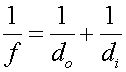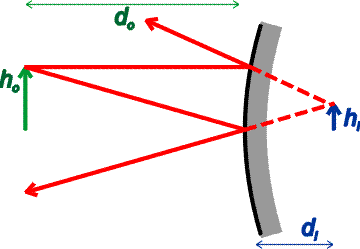Virtual images

For an object far away from a concave mirror, an image appears at the focal point. As the object is moved closer, the image appears further away. Just as the object reaches the focal point, the image has moved all the way to infinity. If you bring the object even closer to the concave mirror, i.e., closer than the focal length, the rays from the object diverge so strongly that the mirror can no longer reconverge them. You can see this algebraically by considering the formulaIf do is less than f, the image distance di will be negative. That does not mean that there is no image; it merely means that the image is virtual. That is to say, the light appears to comes from an image behind the mirror. Looking into a mirror in the morning, you see a virtual image of yourself. In fact, a plane mirror acts like a curved mirror with f set to infinity. In that case di is negative. Convex mirrors never focus light back into a point, but instead always give virtual images. In fact for convex mirrors, the focal length is negative and the same formula applies as above. Let's illustrate a virtual image from a convex mirror.Many automobile mirrors are convex to cover more angle than a plane mirror. The images in such mirrors are smaller than images would be in a plane mirror, so they often carry a warning message "Objects may be closer than they appear".

Mirrors index      examples        Lecture index